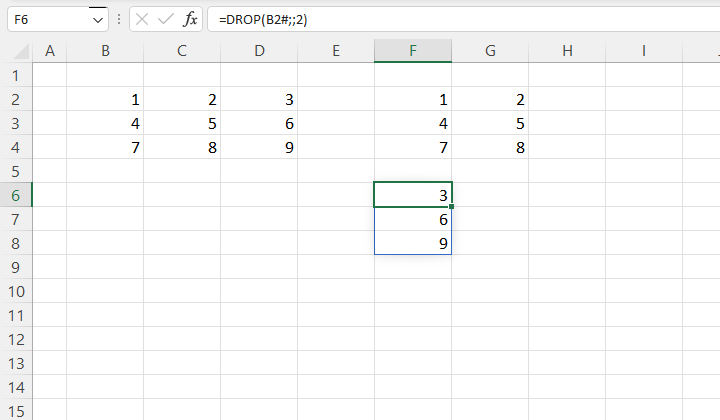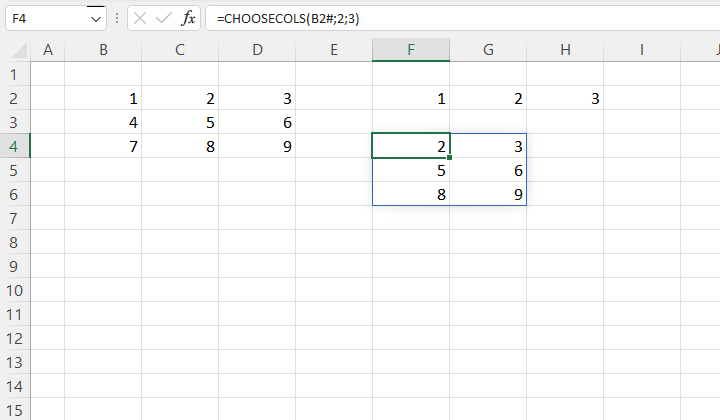If you have used Power Query, you know that it has options that allow you to keep or discard certain columns. If you’re an Excel user coming through a Microsoft 365 subscription, there are a few new functions available to you to do a similar thing. As a result they create a dynamic array with columns or rows extracted from a given matrix that are retained or discarded.

To begin, we’ll use the SEQUENCE function to create a simple matrix containing the numbers 1 through 9. Let’s write the formula:

=SEQUENCE(3,3)

How do we create a new matrix containing only the first two rows? We will use the TAKE function, whose syntax is:

TAKE (<array>,<rows>,[<columns>])

If we write the formula:

=TAKE(B2:D4,,2)

the formula returns a dynamic matrix containing only the first two columns. In this particular case, since the initial matrix was created using the SEQUENCE function, we could also write the formula as follows:

=TAKE(B2#,,2)

If we want to keep only the last column, we should use the DROP function, whose syntax is:

DROP (<array>,<rows>,[<columns>])

Let’s write the formula:

=DROP(B2#,,2)

As a result we will get the last column extracted from the initial matrix.If you explicitly want to set the criteria which row or column should be kept, you can use the CHOOSEROWS or CHOOSECOLS functions. Their syntax:

CHOOSEROWS (<array>,<row 1>,[<row 2>])

CHOOSECOLS (<array>,<col 1>,[<col 2>])

For example, if we want to keep only the first row of the initial matrix, we will write the formula:

=CHOOSEROWS(B2#,1)

Both of these functions allow you to keep multiple rows or columns. For example. if we want to keep the second and third columns, we will write the formula:

=CHOOSECOLS(B2#,2,3)If we want to expand the initial matrix, we will use the EXPAND function. Its syntax: